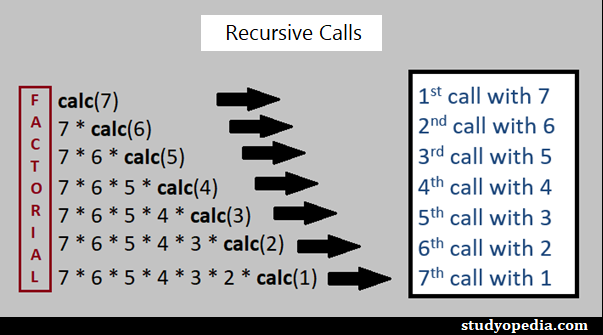## 21 Jan C++ Recursion

In C++, when a function calls itself, it is called Recursion. In another sense, with Recursion, a defined function can call itself. Recursion is a programming approach, which makes code efficient and reduces LOC.

The following figure demonstrates how recursion works when we calculate Factorial in C++ with Recursion:## Recursion Example in C++

Let us now see how to find the factorial of a number in C++ with Recursion:

Output

If you liked the tutorial, spread the word and share the link and our website Studyopedia with others.